# How to Calculate and Solve for Reynold’s Number at Minimum Fluidization | Fluidization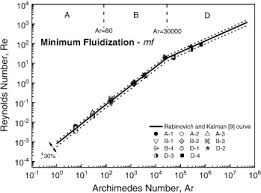The image above represents reynold’s number.

To compute for reynold’s number, four essential parameters are needed and these parameters are Fluidization at Minimum Velocity (umf), Diameter of Bed (d), Density (ρ) and Viscosity (μ).

The formula for calculating reynold’s number:

Re’mf = umf/μ

Where:

Re’mf = Reynold’s Number at Minimum Fluidization
umf = Fluidization at Minimum Velocity
d = Diameter of Bed
ρ = Density
μ = Viscosity

Let’s solve an example;
Find the reynold’s number at minimum fluidization when the fluidization at minimum is 14, the diameter of bed is 8, the density is 12 and the viscosity is 2.

This implies that;

umf = Fluidization at Minimum Velocity = 14
d = Diameter of Bed = 8
ρ = Density = 12
μ = Viscosity = 2

Re’mf = umf/μ
Re’mf = (14)(8)(12)/2
Re’mf = (1344)/2
Re’mf = 672

Therefore, the reynold’s number at minimum fluidization is 672.

Calculating the Fluidization at Minimum Velocity when the Reynold’s Number at Minimum Fluidization, the Diameter of Bed, the Density and the Viscosity is Given.

umf = Re’mf x μ /

Where;

umf = Fluidization at Minimum Velocity
Re’mf = Reynold’s Number at Minimum Fluidization
d = Diameter of Bed
ρ = Density
μ = Viscosity

Let’s solve an example;
Find the fluidization at minimum velocity when the reynold’s number at minimum fluidization is 12, the diameter of bed is 4, the density is 8 and the viscosity is 3.

This implies that;

Re’mf = Reynold’s Number at Minimum Fluidization = 12
d = Diameter of Bed = 4
ρ = Density = 8
μ = Viscosity = 3

umf = Re’mf x μ /
umf = 12 x 3 / (4)(8)
umf = 36 / 32
umf = 1.125

Therefore, the fluidization at minimum velocity is 1.125.

Calculating the Diameter of Bed when the Reynold’s Number at Minimum Fluidization, the Fluidization at Minimum Velocity, the Density and the Viscosity is Given.

d = Re’mf x μ / umfρ

Where;

d = Diameter of Bed
Re’mf = Reynold’s Number at Minimum Fluidization
umf = Fluidization at Minimum Velocity
ρ = Density
μ = Viscosity

Let’s solve an example;
Find the diameter of bed when the reynold’s number at minimum fluidization is 21, the fluidization at minimum velocity is 24, the density is 5 and the viscosity is 7.

This implies that;

Re’mf = Reynold’s Number at Minimum Fluidization = 21
umf = Fluidization at Minimum Velocity = 24
ρ = Density = 5
μ = Viscosity = 7

d = Re’mf x μ / umfρ
d = 21 x 7 / (24)(5)
d = 147 / 120
d = 1.225

Therefore, the diameter of bed is 1.225.

Calculating the Density when the Reynold’s Number at Minimum Fluidization, the Fluidization at Minimum Velocity, the Diameter of Bed and the Viscosity is Given.

ρ = Re’mf x μ / umfd

Where;

ρ = Density
Re’mf = Reynold’s Number at Minimum Fluidization
umf = Fluidization at Minimum Velocity
d = Diameter of Bed
μ = Viscosity

Let’s solve an example;
Find the density when the reynold’s number at minimum fluidization is 8, the fluidization at minimum velocity is 3, the diameter of bed is 10 and the viscosity is 12.

This implies that;

Re’mf = Reynold’s Number at Minimum Fluidization = 8
umf = Fluidization at Minimum Velocity = 3
d = Diameter of Bed = 10
μ = Viscosity = 12

ρ = Re’mf x μ / umfd
ρ = 8 x 12 / (3)(10)
ρ = 96 / 30
ρ = 3.2

Therefore, the density is 3.2.

Calculating the Viscosity when the Reynold’s Number at Minimum Fluidization, the Fluidization at Minimum Velocity, the Diameter of Bed and the Density is Given.

μ = umf / Re’mf

Where;

μ = Viscosity
Re’mf = Reynold’s Number at Minimum Fluidization
umf = Fluidization at Minimum Velocity
d = Diameter of Bed
ρ = Density

Let’s solve an example;
Find the viscosity when the reynold’s number at minimum fluidization is 4, the fluidization at minimum velocity is 8, the diameter of bed is 2 and the density is 6.

This implies that;

Re’mf = Reynold’s Number at Minimum Fluidization = 4
umf = Fluidization at Minimum Velocity = 8
d = Diameter of Bed = 2
ρ = Density = 6

μ = umf / Re’mf
μ = (8)(2)(6) / 4
μ = 96 / 4
μ = 24

Therefore, the viscosity is 24.

Nickzom Calculator – The Calculator Encyclopedia is capable of calculating the reynold’s number at minimum fluidization.

To get the answer and workings of the reynold’s number at minimum fluidization using the Nickzom Calculator – The Calculator Encyclopedia. First, you need to obtain the app.

You can get this app via any of these means:

To get access to the professional version via web, you need to register and subscribe for NGN 2,000 per annum to have utter access to all functionalities.
You can also try the demo version via https://www.nickzom.org/calculator

Apple (Paid) – https://itunes.apple.com/us/app/nickzom-calculator/id1331162702?mt=8
Once, you have obtained the calculator encyclopedia app, proceed to the Calculator Map, then click on Materials and Metallurgical under Engineering.Now, Click on Fluidization under Materials and MetallurgicalNow, Click on Reynold’s Number at Minimum Fluidization under Fluidization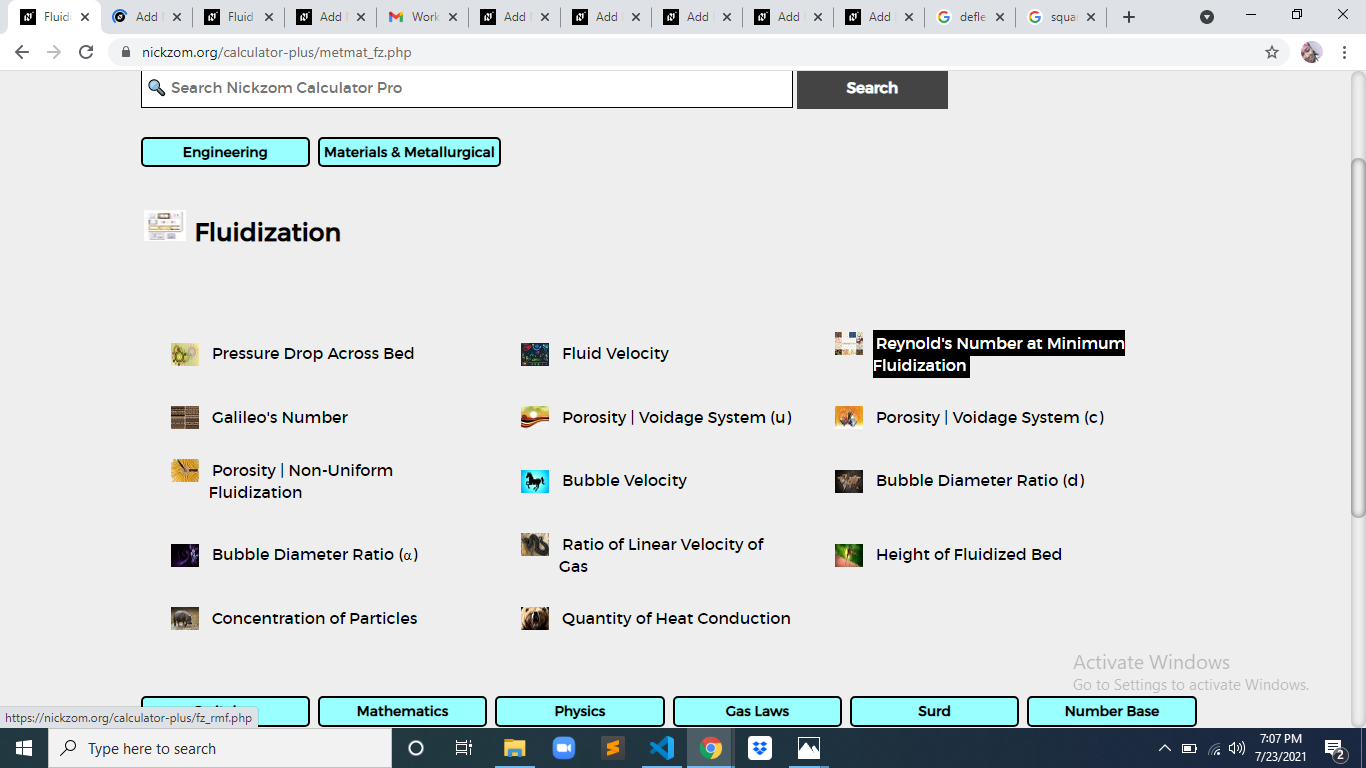The screenshot below displays the page or activity to enter your values, to get the answer for the reynold’s number at minimum fluidization according to the respective parameter which is the Fluidization at Minimum Velocity (umf), Diameter of Bed (d), Density (ρ) and Viscosity (μ).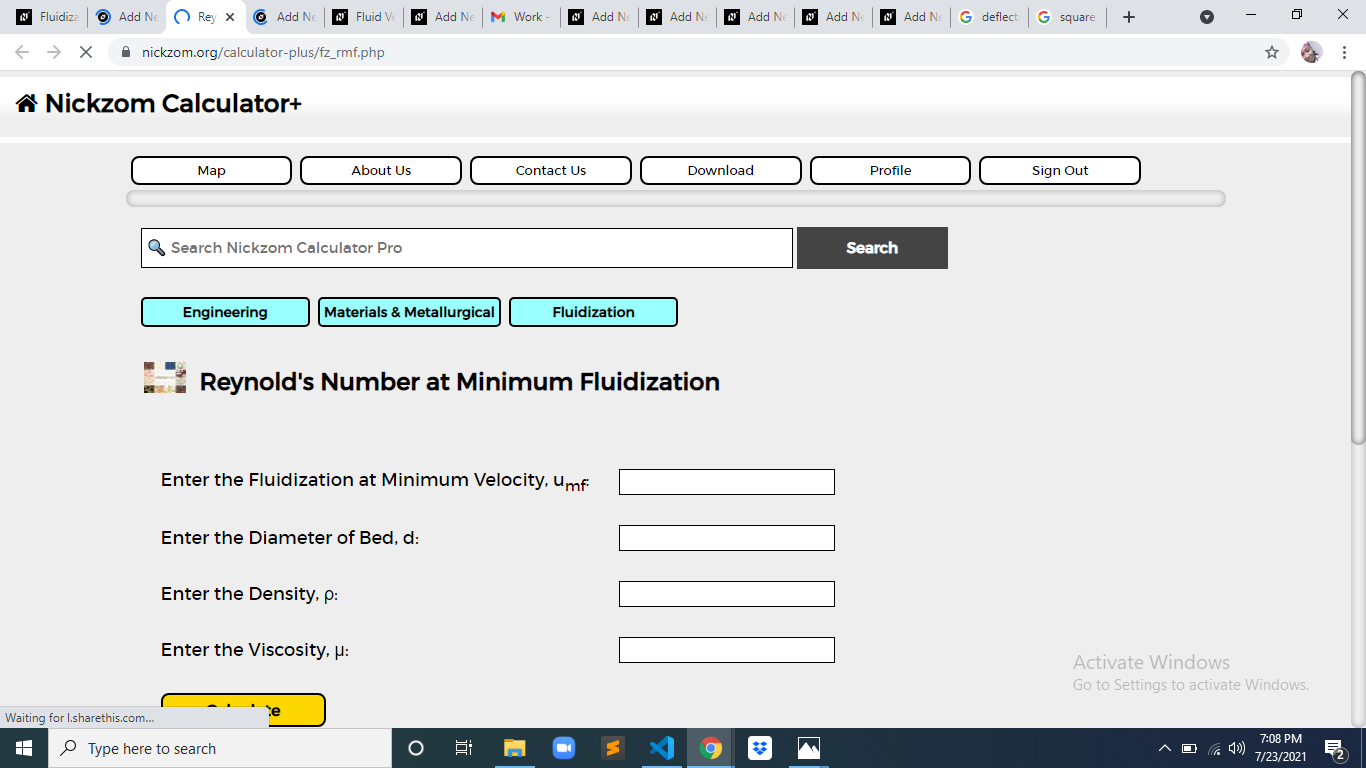Now, enter the values appropriately and accordingly for the parameters as required by the Fluidization at Minimum Velocity (umf) is 8, Diameter of Bed (d) is 8, Density (ρ) is 12 and Viscosity (μ) is 2.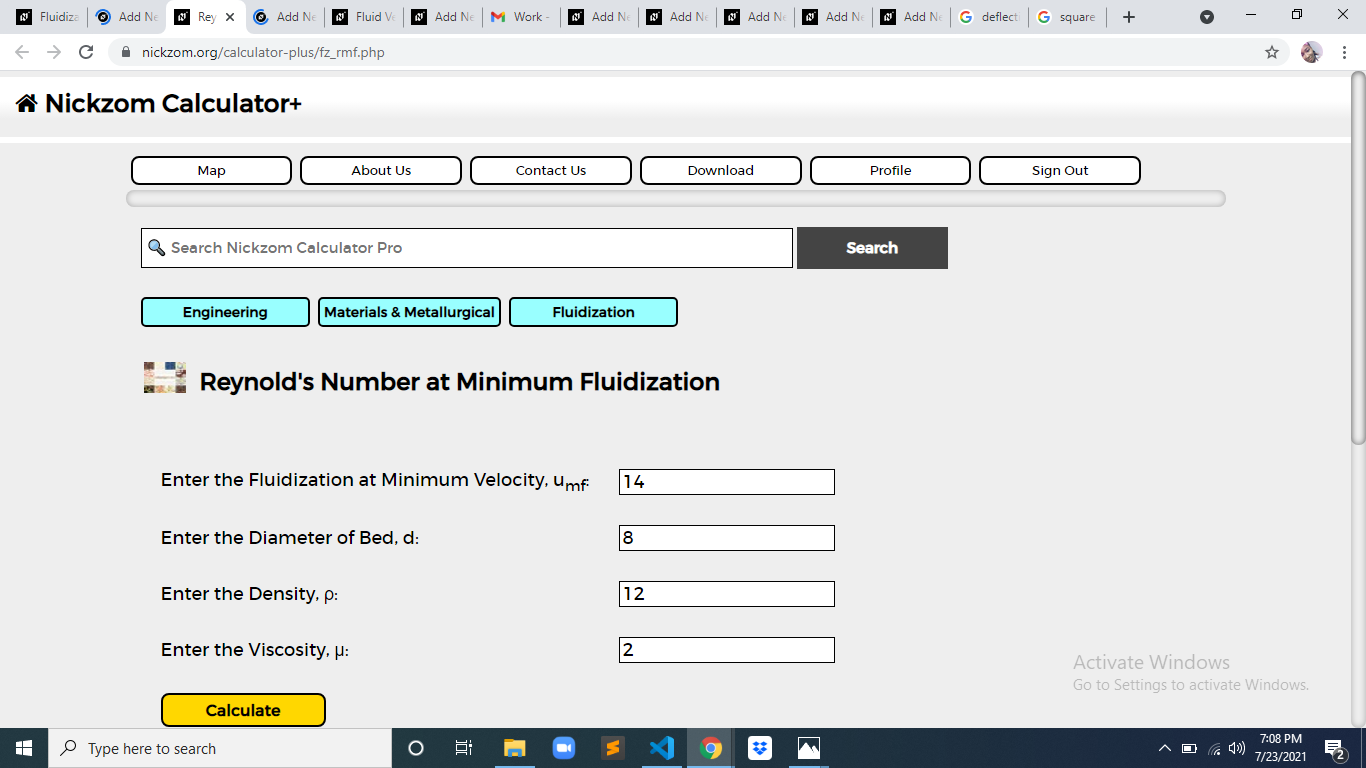Finally, Click on Calculate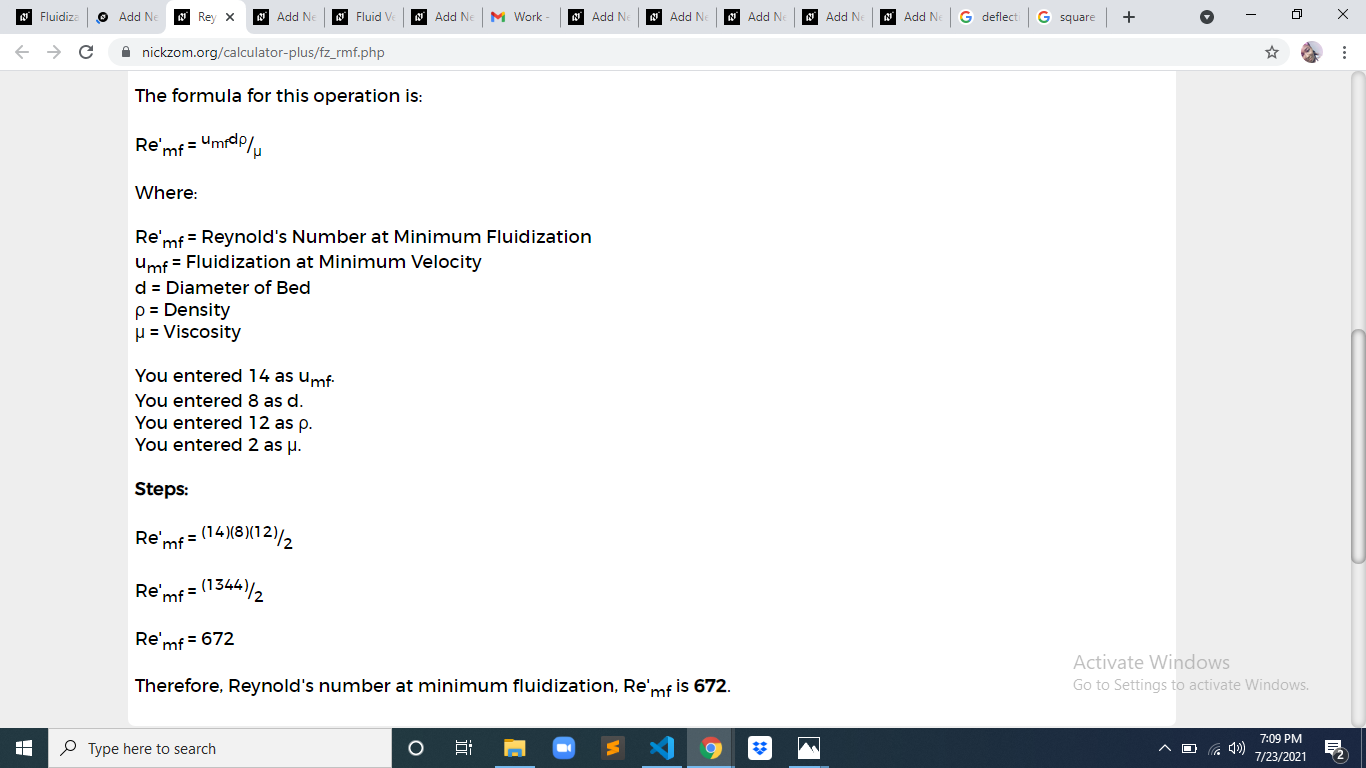As you can see from the screenshot above, Nickzom Calculator– The Calculator Encyclopedia solves for the reynold’s number at minimum fluidization and presents the formula, workings and steps too.